>

# Triangles Enumeration & Counting

## (closed) 5 One Person Data Moving Plug-in licenses to the first 5 that solve the problem below.

Whenever we execute a foreach loop we enumerate all objects that satisfy a given condition (the predicate that defined the set to enumerate). Thus, enumeration plays a foundamental role in computer programming...However, some objects are quite difficult to enumerate!

For instance, try to enumerate all triangles in the picture below: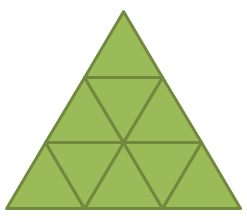9 small triangles plus the triangles below: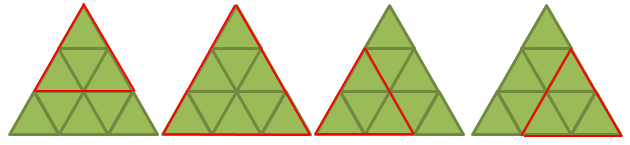For a total of 13.

However, are we sure we enumerated all triangles without missing any of them? Are we sure we avoided duplications(processing the same triangle several time)?

Completeness, and duplication avoidance are the main challanges in most of the enumeration problems!

Let consider now the general problem: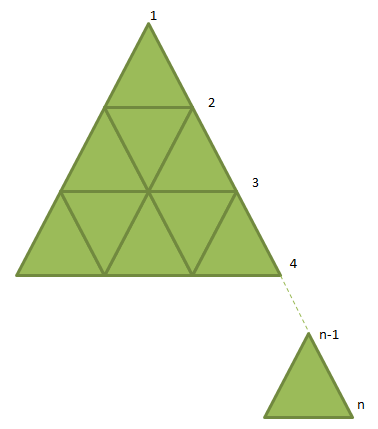n, the number of vertices on each triangle side, is the dimension of the problem.

Since the small triangles are (n-1)(n-1) the computational effort needed to enumerate all triangles must be kn*n, since the number of iterations to enumerate them must be greater than (n-1)(n-1).

However, is it possible to count all triangles (without enumerating them) with the lower computational effort: k'n(linear complexity)?

2. ## Provide an efficient kn algorithm to count them. The algorithm receives as input just n (not all triangle data) and returns the number of triangles, without enumerating all triangles (actually it performs a purely mathematical processing on n).  Explain why the algorithm is supposed to work properly.

The two algorithms may be written in simple text format as instructions for an human operator that must perform the two tasks manually.

The first 5 that will provide a correct solution will win a one person Data Moving Plug-in license and will have their name and curriculum listed in an hall of fame.

Send solutions to [email protected].

Hint:  Please don't forget to include big upside down triangles like the obe in red below: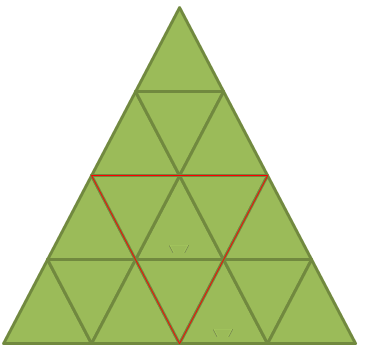They start appearing with a side dimension of 4 (just one). When the side dimension is increaset to 5 they become tree...and so on.

Hint: how to represent data. Vertices are represented by a class with the X and Y vertex coordinates and 6 pointers to its neighbor vertices(some pointer may be null):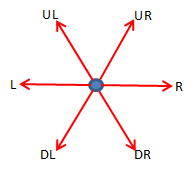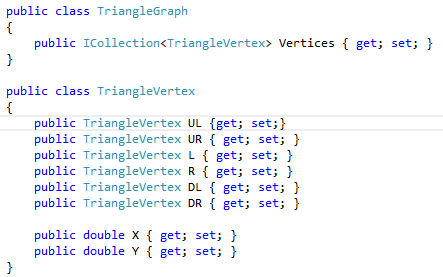Output triangles (the one we are required to enumerate) may be represented with the class: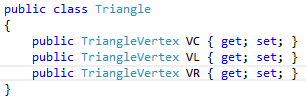Where VL and VR are the respectively the left and right vertices that define the horizontal triangle side and VC is the other vertex:Below also a possible way to implement a constructor of TriangleGraph, that builds a complete triangle graphs of dimension n, where n is the number of vertices on each graph side. This means that for a graph composed of a single small triangle n=2. If you use the different convention of counting the arcs on each graph side, a graph composed of single small triangle would have n=1, instead. You may move from a convention to the other by simply adding/subtracting 1.

The code below compute the proper pointers and coordinates of each vertex graph. You may use it to build a graph to experiment with your counting and enumeration algorithms.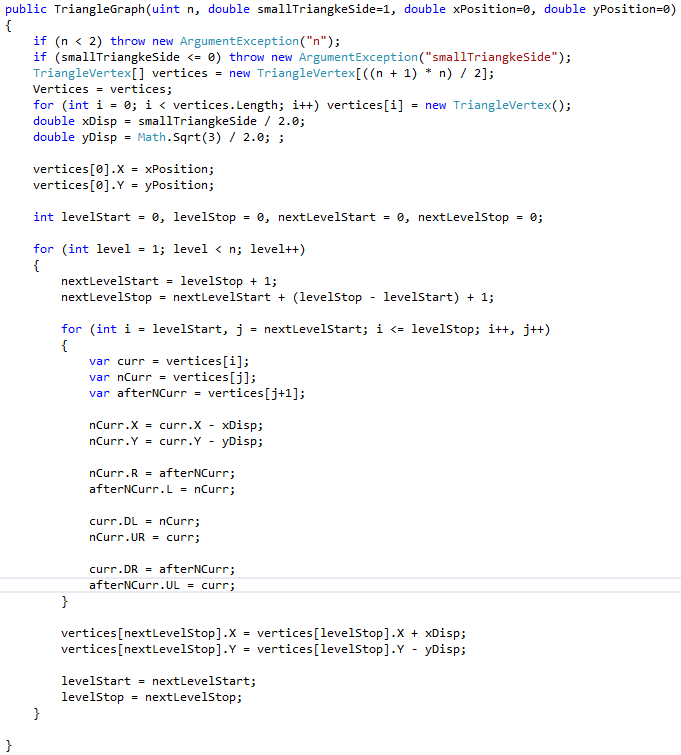PS: You will not find the solution of this problem in any book, blog, artcicle, etc.